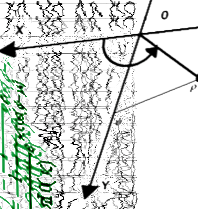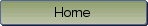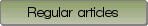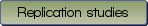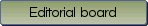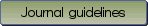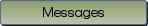Adding bias to reduce variance in psychological results: A tutorial on penalized regression

Bibliographic information: BibTEX format RIS format XML format APA style
Cited references information: BibTEX format APA style

Helwig, Nathaniel E.
1-19
Keywords: penalized least squares , ordinary least squares , ridge , lasso , elastic net
Tools: R
(no sample data)   (Appendix)

Regression models are commonly used in psychological research. In most studies, regression coefficients are estimated via maximum likelihood (ML) estimation. It is well-known that ML estimates have desirable large sample properties, but are prone to overfitting in small to moderate sized samples. In this paper, we discuss the benefits of using penalized regression, which is a form of penalized likelihood (PL) estimation. Informally, PL estimation can be understood as introducing bias to estimators for the purpose of reducing their variance, with the ultimate goal of providing better solutions. We focus on the Gaussian regression model, where ML and PL estimation reduce to ordinary least squares (OLS) and penalized least squares (PLS) estimation, respectively. We cover classic OLS and stepwise regression, as well as three popular penalized regression approaches: ridge regression, the lasso, and the elastic net. We compare the different penalties (or biases) imposed by each method, and discuss the resulting features each penalty encourages in the solution. To demonstrate the methods, we use an example where the goal is to predict a student's math exam performance from 30 potential predictors. Using a step-by-step tutorial with R code, we demonstrate how to (i) load and prepare the data for analysis, (ii) fit the OLS, stepwise, ridge, lasso, and elastic net models, (iii) extract and compare the model fitting results, and (iv) evaluate the performance of each method. Our example reveals that penalized regression methods can produce more accurate and more interpretable results than the classic OLS and stepwise regression solutions.

Be informed of the upcoming issues with RSS feed:RSS